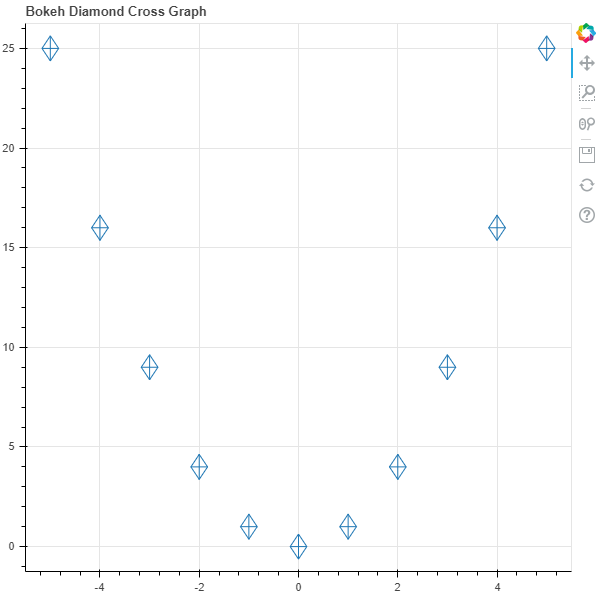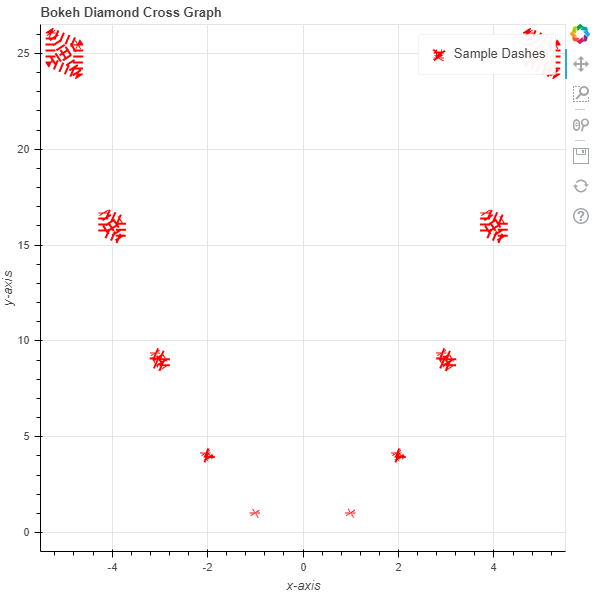# Python Bokeh – Plotting Diamond Crosses on a Graph

Bokeh is a Python interactive data visualization. It renders its plots using HTML and JavaScript. It targets modern web browsers for presentation providing elegant, concise construction of novel graphics with high-performance interactivity.

Bokeh can be used to plot diamond with crosses on a graph. Plotting diamond with crosses on a graph can be done using the `diamond_cross()` method of the `plotting` module.

## plotting.figure.diamond_cross()

Syntax : diamond_cross(parameters)

Parameters :

• x : x-coordinates of the center of the diamond cross markers
• y : y-coordinates of the center of the diamond cross markers
• size : diameter of the diamond cross markers, default is 4
• angle : angle of rotation of the diamond cross markers, default is 0
• angle_units : unit of the angle, default is rad
• fill_alpha : fill alpha value of the diamond cross markers
• fill_color : fill color value of the diamond cross markers
• line_alpha : percentage value of line alpha, default is 1
• line_cap : value of line cap for the line, default is butt
• line_color : color of the line, default is black
• line_dash : value of line dash such as :
• solid
• dashed
• dotted
• dotdash
• dashdot

default is solid

• line_dash_offset : value of line dash offset, default is 0
• line_join : value of line join, default in bevel
• line_width : value of the width of the line, default is 1
• name : user-supplied name for the model
• tags : user-supplied values for the model

Other Parameters :

• alpha : sets all alpha keyword arguments at once
• color : sets all color keyword arguments at once
• legend_field : name of a column in the data source that should be used
• legend_group : name of a column in the data source that should be used
• legend_label : labels the legend entry
• muted : determines whether the glyph should be rendered as muted or not, default is False
• name : optional user-supplied name to attach to the renderer
• source : user-supplied data source
• view : view for filtering the data source
• visible : determines whether the glyph should be rendered or not, default is True
• x_range_name : name of an extra range to use for mapping x-coordinates
• y_range_name : name of an extra range to use for mapping y-coordinates
• level : specifies the render level order for this glyph

Returns : an object of class `GlyphRenderer`

Example 1 :In this example we will be using the default values for plotting the graph. We have provided the size and fill_color attributes to make the glyph visible.

 `# importing the modules ` `from` `bokeh.plotting ``import` `figure, output_file, show ` `    `  `# file to save the model ` `output_file(``"gfg.html"``) ` `    `  `# instantiating the figure object ` `graph ``=` `figure(title ``=` `"Bokeh Diamond Cross Graph"``) ` `  `  `# the points to be plotted ` `x ``=` `[``-``5``, ``-``4``, ``-``3``, ``-``2``, ``-``1``, ``0``, ``1``, ``2``, ``3``, ``4``, ``5``] ` `y ``=` `[i ``*``*` `2` `for` `i ``in` `x] ` ` `  `# plotting the graph ` `graph.diamond_cross(x, y, size ``=` `25``, fill_color ``=` `None``) ` `  `  `# displaying the model ` `show(graph) `

Output :Example 2 :In this example we will be plotting the diamond crossess with dotted lines alongside other parameters and the size of the diamond crosses are in proportion to their values.

 `# importing the modules ` `from` `bokeh.plotting ``import` `figure, output_file, show ` `    `  `# file to save the model ` `output_file(``"gfg.html"``) ` `    `  `# instantiating the figure object ` `graph ``=` `figure(title ``=` `"Bokeh Diamond Cross Graph"``) ` `  `  `# name of the x-axis ` `graph.xaxis.axis_label ``=` `"x-axis"` `   `  `# name of the y-axis ` `graph.yaxis.axis_label ``=` `"y-axis"` `  `  `# the points to be plotted ` `x ``=` `[``-``5``, ``-``4``, ``-``3``, ``-``2``, ``-``1``, ``0``, ``1``, ``2``, ``3``, ``4``, ``5``] ` `y ``=` `[i ``*``*` `2` `for` `i ``in` `x] ` `  `  `# size of the diamonds ` `size ``=` `[i ``*` `2` `for` `i ``in` `y] ` `  `  `# angle of the diamonds ` `angle ``=` `10` ` `  `# fill color value ` `fill_color ``=` `None` ` `  `# color of the line ` `line_color ``=` `"red"` `   `  `# type of line ` `line_dash ``=` `"dotted"` `   `  `# offset of line dash ` `line_dash_offset ``=` `1` `  `  `# width of the dashes ` `line_width ``=` `10` `  `  `# name of the legend ` `legend_label ``=` `"Sample Dashes"` `   `  `# plotting the graph ` `graph.diamond_cross(x, y, ` `                    ``size ``=` `size, ` `                    ``angle ``=` `angle, ` `                    ``fill_color ``=` `fill_color, ` `                    ``line_color ``=` `line_color, ` `                    ``line_dash ``=` `line_dash, ` `                    ``line_dash_offset ``=` `line_dash_offset, ` `                    ``line_width ``=` `line_width, ` `                    ``legend_label ``=` `legend_label) ` `  `  `    `  `# displaying the model ` `show(graph) `

Output :My Personal Notes arrow_drop_upCheck out this Author's contributed articles.

If you like GeeksforGeeks and would like to contribute, you can also write an article using contribute.geeksforgeeks.org or mail your article to contribute@geeksforgeeks.org. See your article appearing on the GeeksforGeeks main page and help other Geeks.

Please Improve this article if you find anything incorrect by clicking on the "Improve Article" button below.

Article Tags :

Be the First to upvote.

Please write to us at contribute@geeksforgeeks.org to report any issue with the above content.